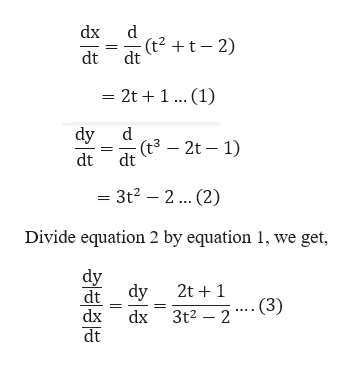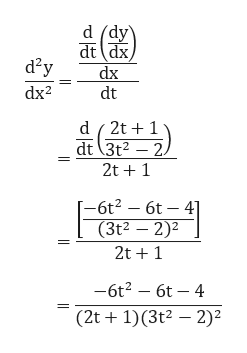# For the curve given by x=t^2+t-2,y=t^3-2t-1, find the slope and concavity at the point (4 , 3).

Question
83 views

For the curve given by x=t^2+t-2,y=t^3-2t-1, find the slope and concavity at the point (4 , 3).

check_circle

Step 1

In order to find the slope at point we need the value of t (the parameter) which is missing in the question. Please verify the question.

Given a curve

Step 2

Now differentiate x and y with respect to t, we get the following result.help_outlineImage Transcriptionclosedx d (t2 +t– 2) dt dt = 2t + 1 ... (1) dy (t³ – 2t – 1) dt dt = 3t2 – 2... (2) Divide equation 2 by equation 1, we get, dy dy 2t + 1 dt - (3) dx dx 3t2 – 2 dt fullscreen
Step 3

For concavity, again, different...help_outlineImage Transcriptionclosed (dy\ dt dx dx d²y dt dx2 d ( 2t + 1 dt (3t2 – 2. 2t + 1 -6t2 – 6t – 41 (3t2 – 2)2 2t +1 -6t2 – 6t – 4 (2t + 1)(3t² – 2)² fullscreen

### Want to see the full answer?

See Solution

#### Want to see this answer and more?

Solutions are written by subject experts who are available 24/7. Questions are typically answered within 1 hour.*

See Solution
*Response times may vary by subject and question.
Tagged in

### Calculus